# Kriging¶

Kriging (also known as Gaussian process regression) is a Bayesian technique that aim at approximating functions (most often in order to surrogate it because it is expensive to evaluate). In the following it is assumed we aim at creating a surrogate model of a scalar-valued model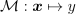. Note the implementation of Kriging deals with vector-valued functions (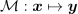), without simply looping over each output. It is also assumed the model is obtained over a design of experiments in order to produce a set of observations gathered in the following dataset: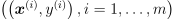. Ultimately Kriging aims at producing a predictor (also known as a response surface or metamodel) denoted as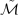.

We put the following Gaussian process prior on the model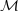: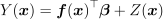where:

•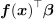is a generalized linear model based upon a functional basis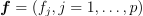and a vector of coefficients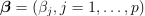,

•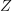is a zero-mean stationary Gaussian process whose covariance function reads: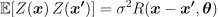where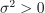is the variance and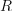is the correlation function that solely depends on the Manhattan distance between input points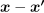and a vector of parameters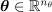.

Under the Gaussian process prior assumption, the observations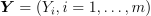and a prediction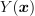at some unobserved inputare jointly normally distributed: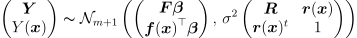where: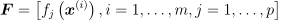is the regression matrix,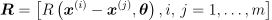is the observations’ correlation matrix, and: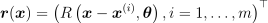is the vector of cross-correlations between the prediction and the observations.

As such, the Kriging predictor is defined as the following conditional distribution: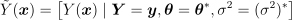where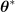and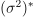are the maximum likelihood estimates of the correlation parameters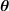and variance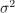(see references).

It can be shown (see references) that the predictor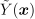is also Gaussian: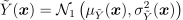• with mean: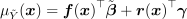where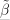is the generalized least squares solution of the underlying linear regression problem: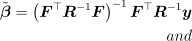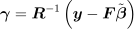• and variance: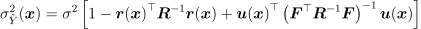where: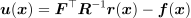Kriging may also be referred to as Gaussian process regression.